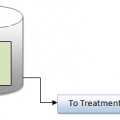## Calculating Calcium Hardness as CaCO3

Calculating Calcium Hardness as CaCO3

The hardness (in mg/L as CaCO3) for any given metallic ion is calculated using Equation

Calcium hardness (m/L) as CaCO3/equivalent weight of CaCO3

= Calcium (mg/L)/equivalent weight of calcium

Example:-

A water sample has calcium content of 51 mg/L. What is this calcium hardness expressed as CaCO3?

Solution
Referring to Equation:

x mg/L /50.045=51 mg/L /20.04

x=51 x 50.045/20.04

=127.36 mg /L Ca as CaCO3

Technorati Tags: water analysis,water hardness## Author:T.P.Sivanandan

#### Why Fine Bubbles are Better than Coarse bubble in Aeration Tank#### How to Calculate Lime Dosage Requirement in Sedimentation Process## 11 thoughts on “Calculating Calcium Hardness as CaCO3”

1.Saravanan says:

50.045 means

1.T.P.Sivanandan says:

Equivalent weight of Calcium Carbonate

2.Eddie Hernandez says:

Frank R. Spellman and it states that the answer is 124.8 mg/L Ca as CaCO3.

Seems to follow the calculation I always knew of 2.5(Ca++) thus 2.5*51=127.5 mg/L
Close enough…….

Who’s right and who’s wrong?

1.T.P.Sivanandan says:

thanks your clarification 124.8 is accuracy fig

3.Rajendra Prajapati says:

Molar mass (CO3(2-)) = M(C) + 3 x M(O) = 12+ 3 x 16 = 60 g/mole. Molar mass (CaCO3) = M(Ca) + M(C) + 3 x M(O) = 40 + 12 + 3 x 16 = 100 g/mole. Divide the molar mass by the ion charge or oxidation number (for CaCO3) to determine equivalent (Eq.) weights. Eq. weight (HCO3(-)) = 61 / 1 (charge) = 61 g/Eq.

So eq wt of Caco3 is 61g/eq it is ok

4.edemita adams says:

Pls can u apply this conversion to to any sample of interest esp soil sample. Hw many unit do you av to subtract to obtain an accurate accuracy figure?.. reply ASAP pls

5.edemita adams says:

And pls whr did u derive 20.04? Pls I need a clearification

6.edemita adams says:

Is it ca3-ca?

7.Ram Bow says:

Calculation above is wrong.

If x=51 x 50.045/20.04,
then x=127.36 mg /L Ca as CaCO3
not x= 124.8!

1.T.P.Sivanandan says:

Thanks to point us, we updated